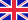•Algebraic topology

Autor: Source: Wikipedia

Source: Wikipedia. Pages: 176. Chapters: Tesseract, Fundamental group, 3-sphere, Polychoron, Borsuk-Ulam theorem, Euler characteristic, Genus, Knot theory, Homological algebra, Winding number, Sheaf, Covering space, Homology theory, Spectral sequence, Abstract... Viac o knihe

Na objednávku, dodanie 2-4 týždne

32.39 €

bežná cena: 38.10 €

O knihe

Source: Wikipedia. Pages: 176. Chapters: Tesseract, Fundamental group, 3-sphere, Polychoron, Borsuk-Ulam theorem, Euler characteristic, Genus, Knot theory, Homological algebra, Winding number, Sheaf, Covering space, Homology theory, Spectral sequence, Abstract polytope, Cobordism, Hopf fibration, Vector bundle, Higher-dimensional algebra, Fiber bundle, Algebraic K-theory, Formal group, Surgery theory, Steenrod algebra, Spin structure, Seifert-van Kampen theorem, Quasitoric manifold, CW complex, Highly structured ring spectrum, List of cohomology theories, Lefschetz hyperplane theorem, Intersection homology, Simplicial set, Nonholonomic system, Twisted K-theory, Line bundle, Associated bundle, Esquisse d'un Programme, Real projective space, Triangulation, Simply connected space, Massey product, Barycentric subdivision, Characteristic class, Complex cobordism, Vietoris-Rips complex, Cech cohomology, Künneth theorem, Clique complex, Duoprism, Abstract simplicial complex, Betti number, Acyclic model, Serre spectral sequence, Simplicial homology, Sheaf cohomology, Monodromy, Landweber exact functor theorem, Delta set, Classifying space, Lefschetz fixed-point theorem, Free product, Cup product, Combinatorial map, Hurewicz theorem, Degree of a continuous mapping, Eilenberg-MacLane space, String topology, Cellular approximation, L-theory, Solenoid, Serre-Swan theorem, Ramification, Calculus of functors, Topological modular forms, Induced homomorphism, Surgery structure set, Thom space, Riemann-Hurwitz formula, Crossed module, Mapping cone, Elliptic cohomology, Topological K-theory, Mapping cylinder, A8-operad, Invariance of domain, Aspherical space, Semi-locally simply connected, Duocylinder, Rose, Lusternik-Schnirelmann category, Homotopy lifting property, E8-operad, R-algebroid, Cap product, Poincaré complex, Microbundle, Semi-s-cobordism, List of algebraic topology topics, Eilenberg-Zilber theorem, Alexander-Spanier cohomology, Cohomology operation, Chain, Alexander duality, Virtual knot, Fundamental class, Godement resolution, Morava K-theory, Gysin sequence, Combinatorial topology, Plus construction, Lie algebra bundle, Universal coefficient theorem, Homotopy extension property, Bockstein homomorphism, Categorification, Equivariant cohomology, H-space, Acyclic space, Euler calculus, Size functor, Spinor bundle, Size homotopy group, Shriek map, Doomsday conjecture, Vanishing cycle, Regular homotopy, Ganea conjecture, Join, Simplex category, Generalized map, Chern-Simons form, Pseudocircle, Adams operation, Brown-Peterson cohomology, Presentation complex, Homotopical algebra, Directed algebraic topology, Facet, N-skeleton, Simplicial approximation theorem, Homology manifold, Homotopy fiber, Homeotopy, Essential manifold, Local system, Whitehead link, Stallings-Zeeman theorem, Leray-Hirsch theorem, Collapse, Simplicial map, Infinite symmetric product, Direct limit of groups, Timelike homotopy, Double torus knot, Eilenberg-Ganea conjecture, Moore space, James reduced product, Vietoris-Begle mapping theorem, Hilton's theorem, Timelike simply connected, Whitehead conjecture, Quasi-isomorphism, Dold-Thom theorem, Topological pair, List of homological algebra topics, Simply connected at infinity, Sphere spectrum, Poincaré space, Inverse bundle, Dold manifold, House with two rooms, Chern-Weil theory, Cartan model, Pair of spaces, Cocycle class, Classifying space for O(n). Excerpt: In mathematics, a sheaf is a tool for systematically tracking locall...

• Vydavateľstvo: Books LLC, Reference Series
• Rok vydania: 2011
• Formát: Paperback
• Rozmer: 246 x 189 mm
• Jazyk: Anglický jazyk
• ISBN: 9781157666004

Generuje redakčný systém BUXUS CMS spoločnosti ui42.